Скачать презентацию Scientific Method Steps in the Scientific Method

scientific_method.ppt

• Размер: 425.5 Кб
• Количество слайдов: 25

## Описание презентации Scientific Method Steps in the Scientific Method по слайдам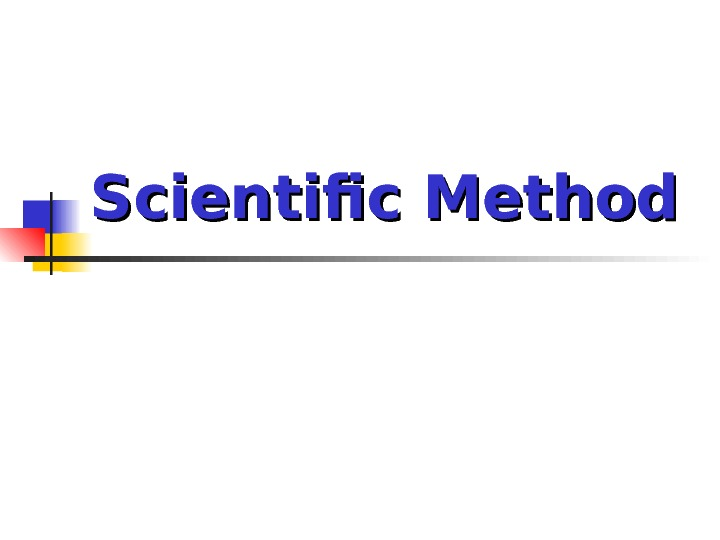Scientific Method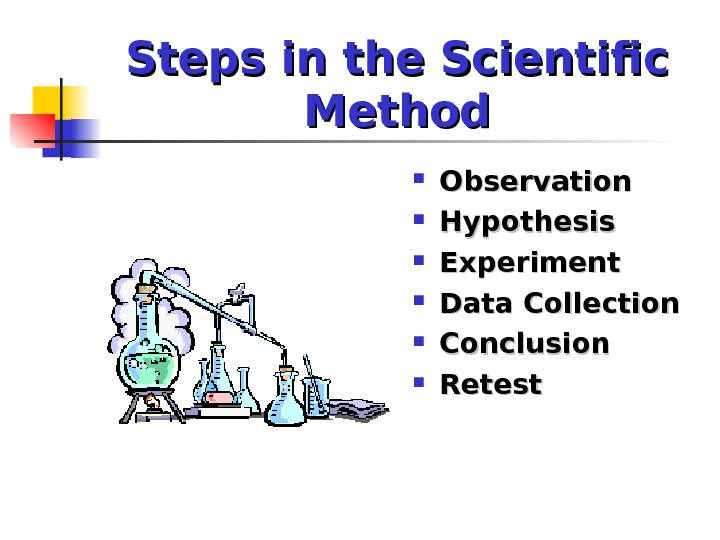Steps in the Scientific Method Observation Hypothesis Experiment Data Collection Conclusion Retest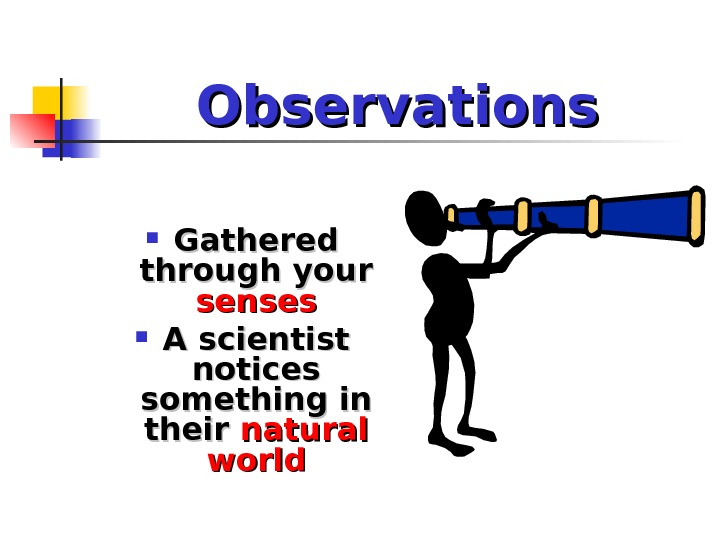Observations Gathered through your senses A scientist notices something in their natural world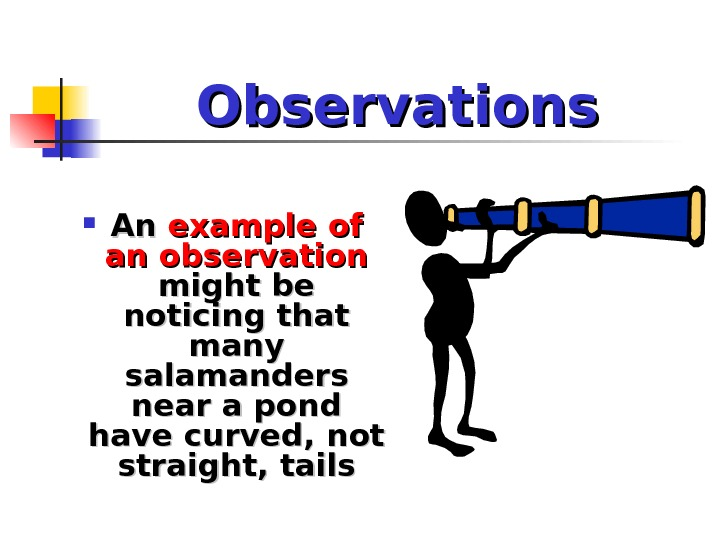Observations An An example of of an observation might be noticing that many salamanders near a pond have curved, not straight, tails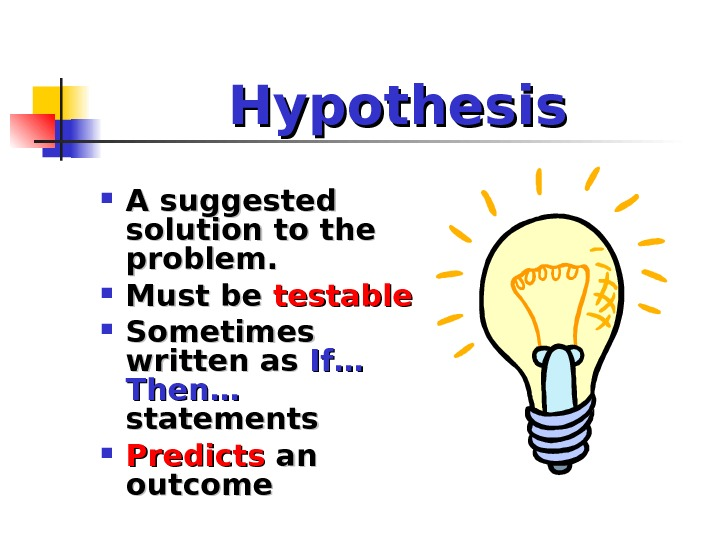Hypothesis A suggested solution to the problem. Must be testable Sometimes written as If…If… Then… statements Predicts an an outcome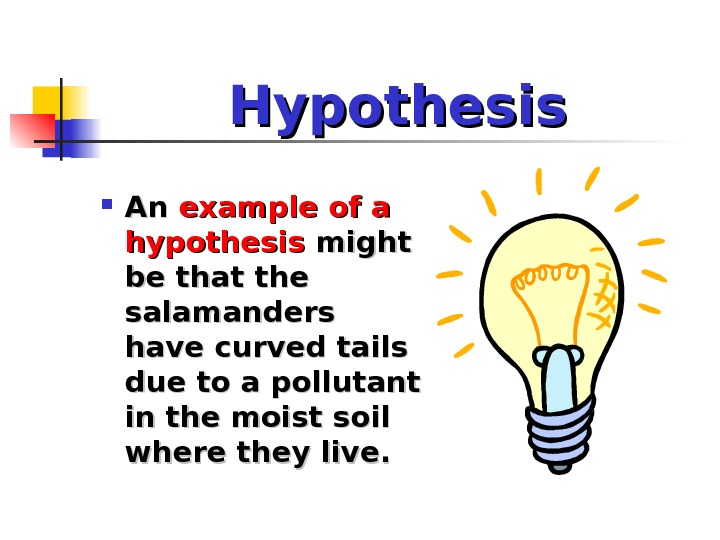Hypothesis An An example of a hypothesis might be that the salamanders have curved tails due to a pollutant in the moist soil where they live.Experiment A A procedure to to test the hypothesis. .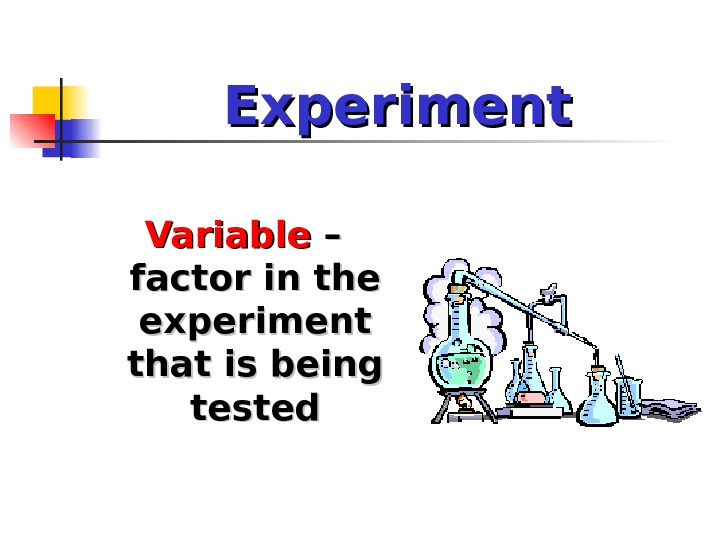Experiment Variable – – factor in the experiment that is being tested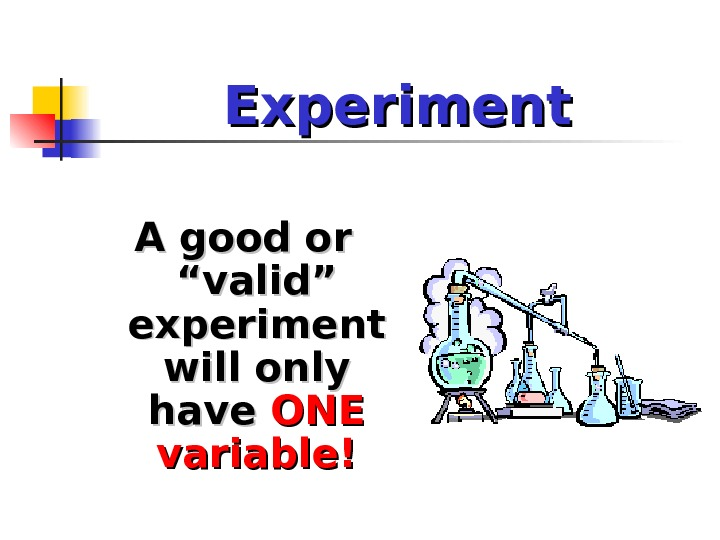Experiment A good or “valid” experiment will only have ONE variable!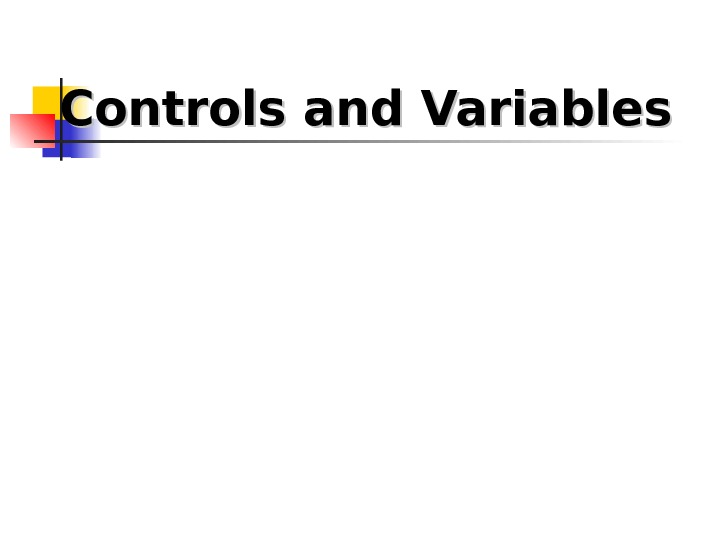Controls and Variables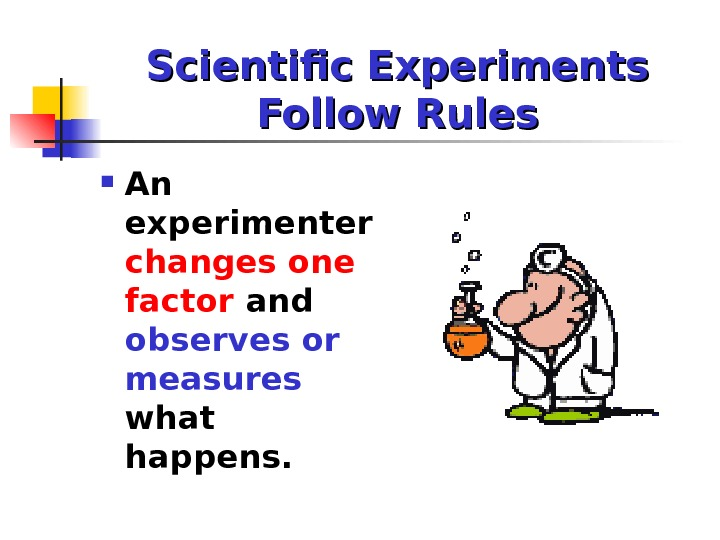Scientific Experiments Follow Rules An experimenter changes one factor and observes or measures what happens.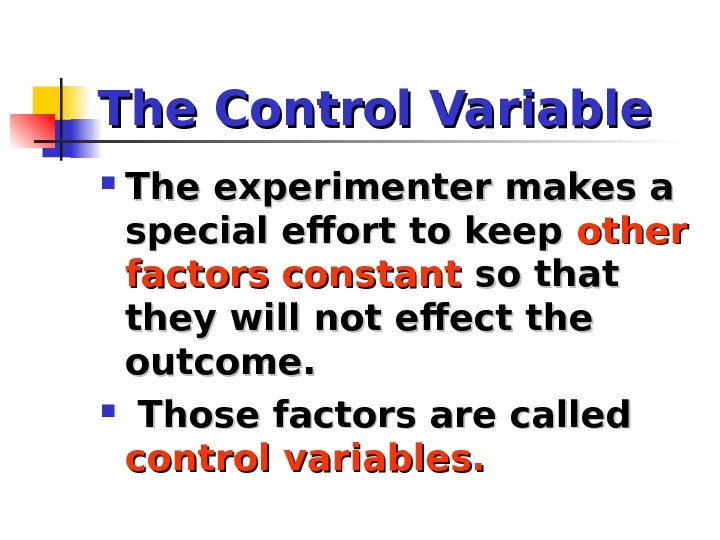The Control Variable The experimenter makes a special effort to keep other factors constant so that they will not effect the outcome. Those factors are called control variables.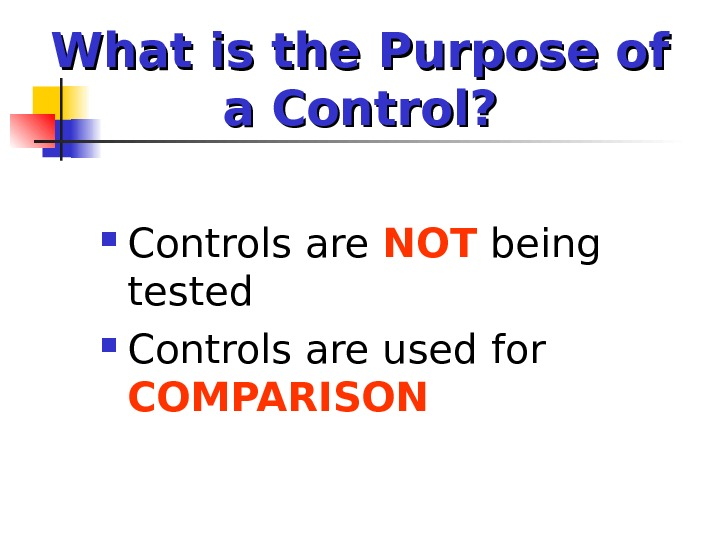What is the Purpose of a Control? Controls are NOT being tested Controls are used for COMPARISON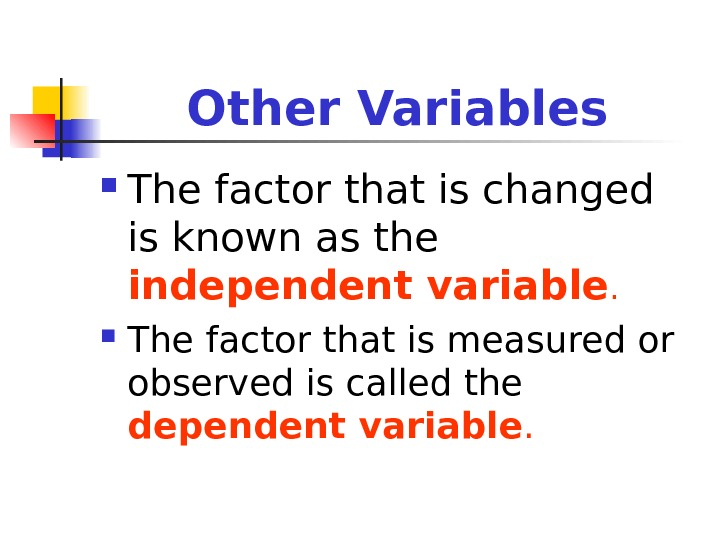Other Variables The factor that is changed is known as the independent variable. The factor that is measured or observed is called the dependent variable.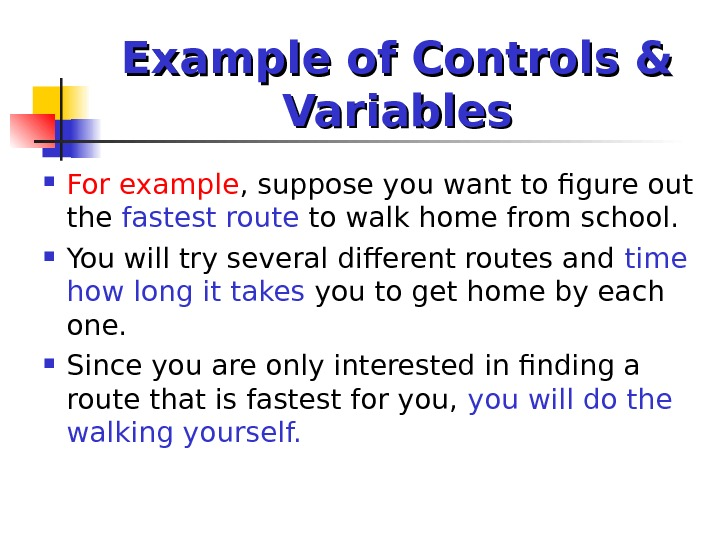Example of Controls & Variables For example , suppose you want to figure out the fastest route to walk home from school. You will try several different routes and time how long it takes you to get home by each one. Since you are only interested in finding a route that is fastest for you, you will do the walking yourself.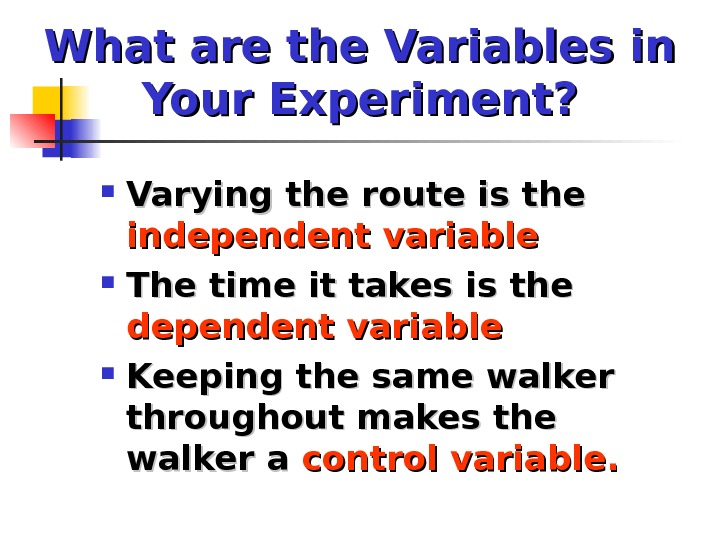What are the Variables in Your Experiment? Varying the route is the independent variable The time it takes is the dependent variable Keeping the same walker throughout makes the walker a control variable.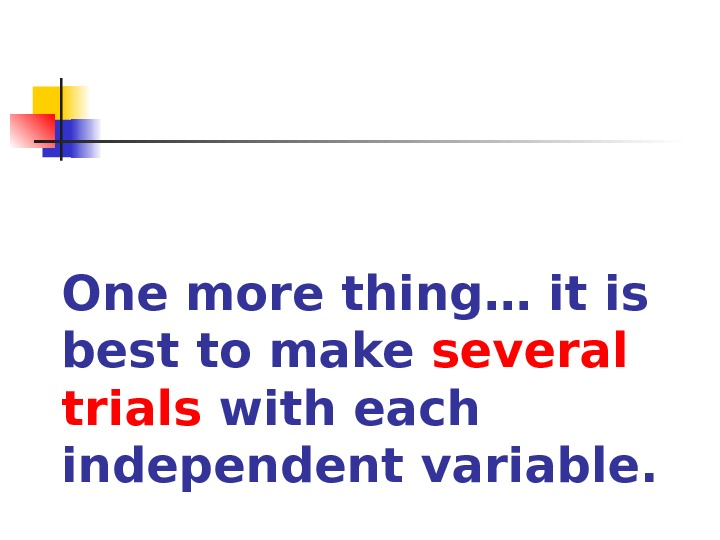One more thing… it is best to make several trials with each independent variable.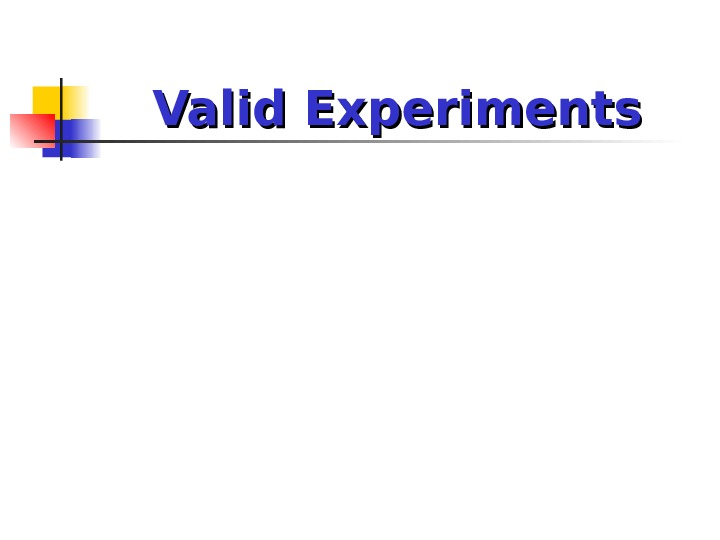Valid Experiments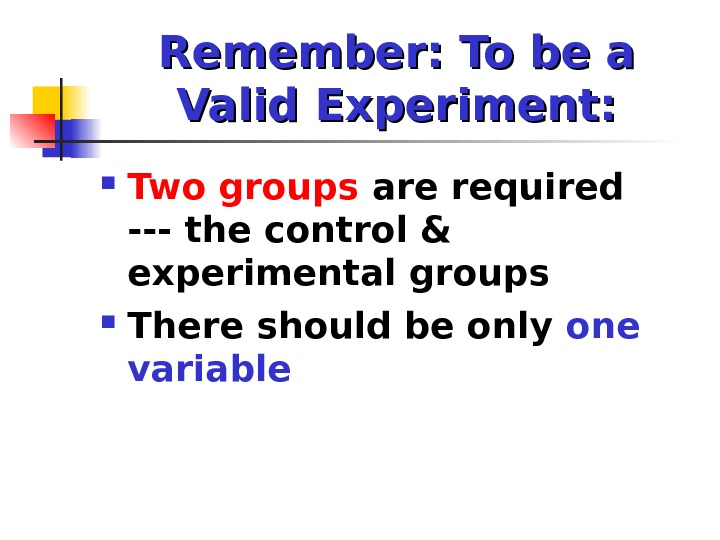Remember: To be a Valid Experiment: Two groups are required — the control & experimental groups There should be only one variable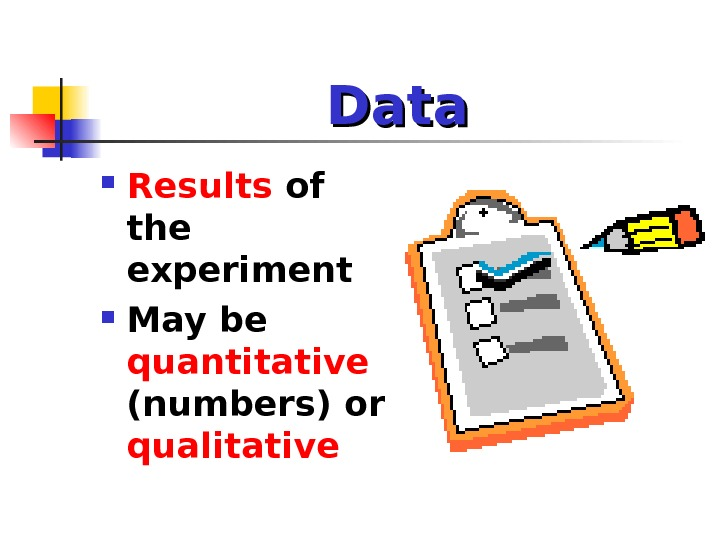Data Results of the experiment May be quantitative (numbers) or qualitative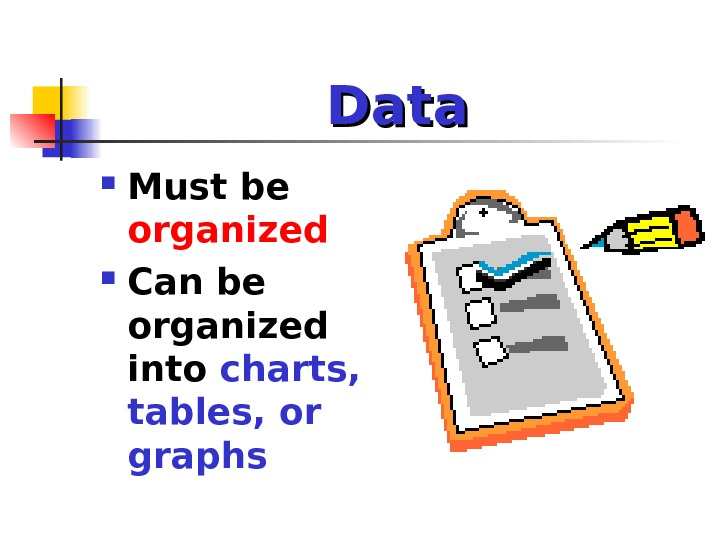Data Must be organized Can be organized into charts, tables, or graphs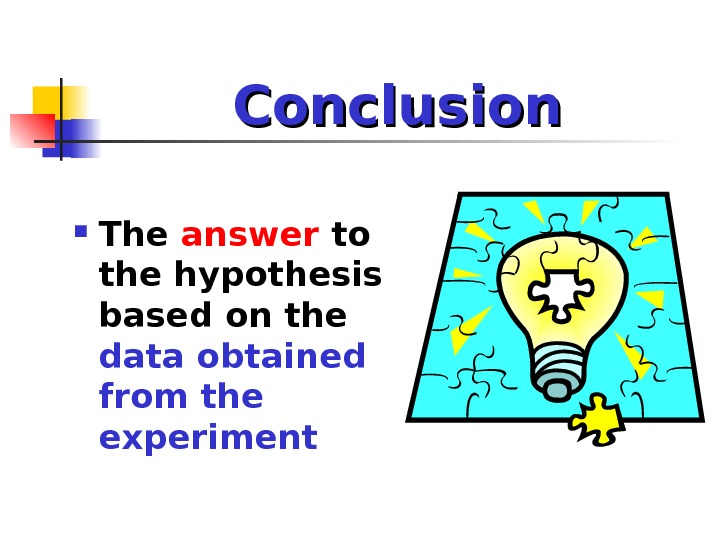Conclusion The answer to the hypothesis based on the data obtained from the experiment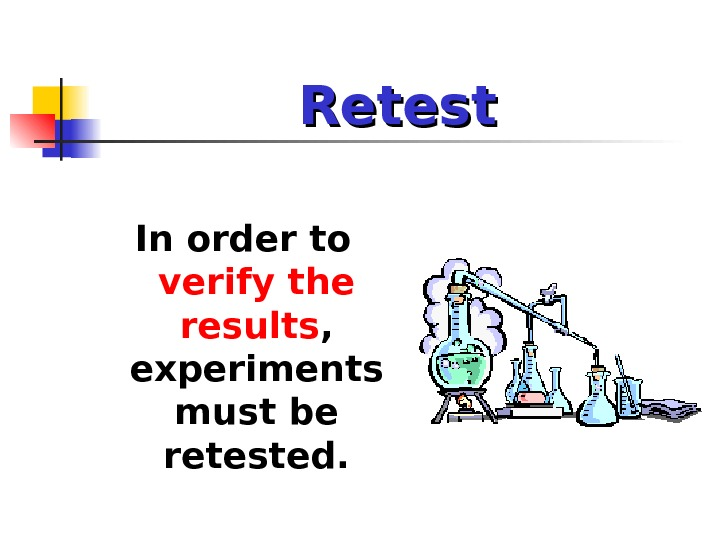Retest In order to verify the results , experiments must be retested.Review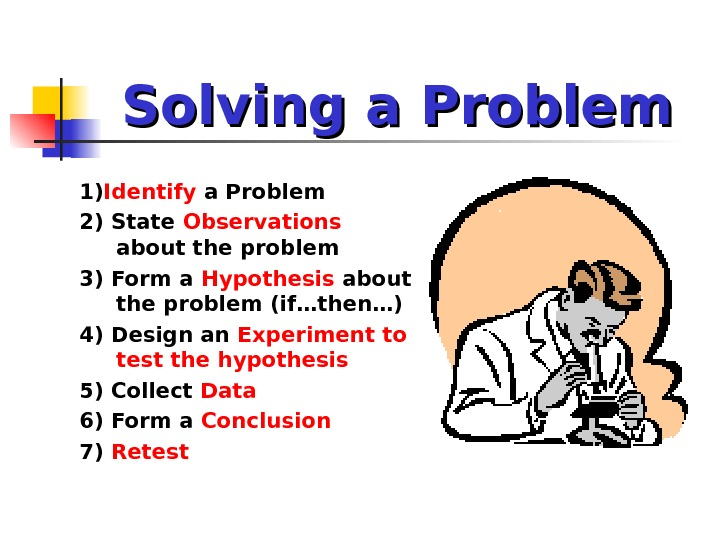Solving a Problem 1) Identify a Problem 2) State Observations about the problem 3) Form a Hypothesis about the problem (if…then…) 4) Design an Experiment to test the hypothesis 5) Collect Data 6) Form a Conclusion 7) Retest